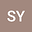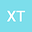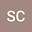One-dimensional periodic fractional Schrödinger equations with exponential critical growth
•••Xianhua Tang
Central South University
Author ProfileSitong Chen
Central South University
Author Profile## Abstract

In the present paper, we study the existence of nontrivial solutions of the following one-dimensional fractional Schr\“{o}dinger equation $$(-\Delta)^{1/2}u+V(x)u=f(x,u), \ \ x\in \R,$$ where $(-\Delta)^{1/2}$ stands for the $1/2$-Laplacian, $V(x)\in \mathcal{C}(\R, (0,+\infty))$, and $f(x,u):\R\times\R\to \R$ is a continuous function with an exponential critical growth. Comparing with the existing works in the field of exponential-critical-growth fractional Schr\”{o}dinger equations, we encounter some new challenges due to the weaker assumptions on the reaction term $f$. By using some sharp energy estimates, we present a detailed analysis of the energy level, which allows us to establish the existence of nontrivial solutions for a wider class of nonlinear terms. Furthermore, we use the non-Nehari manifold method to establish the existence of Nehari-type ground state solutions of the one-dimensional fractional Schr\”{o}dinger equations.
21 Dec 2021Submitted to Mathematical Methods in the Applied Sciences
22 Dec 2021Submission Checks Completed
22 Dec 2021Assigned to Editor
10 Jan 2022Reviewer(s) Assigned
19 Apr 2022Review(s) Completed, Editorial Evaluation Pending
18 May 2022Editorial Decision: Revise Minor